Prev: 2.3.2 Line Elements Up: 2.3 Optimizations for the Next: 3. Solid Modeling and

## 2.3.3 Benchmark Example and Run Time Considerations

With the three algorithms described above, namely, the original sphere algorithm (a) from Fig. 2.3(a), the spherical segments algorithm (b) from Fig. 2.3(b) in Section 2.3.1, and the line algorithm (c) from Section 2.3.2, simulations of isotropic deposition of aluminium into a 0.5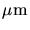diameter cylindrical via were carried out. The initial geometry and the film profiles after the two time-steps can be seen in Fig. 2.6. For more clarity only a quarter of the structure is shown.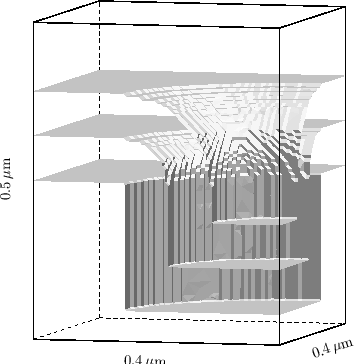The deposition rate was 0.07/s and the deposition time 120s. In total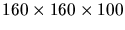cells were used for the simulation domain of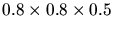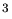. The simulation was performed with two time-steps of 60s. All calculations were done on a 200MHz DEC Alpha workstation.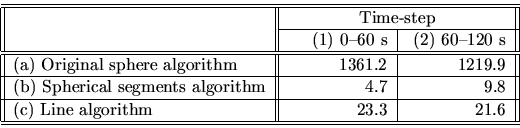Table 2.1 summarizes the calculation times obtained with the different algorithms. Two major observations are obtainable from the figures: Firstly, both spherical segments (b) as well as line elements (c) lead to an acceleration in the range of two orders of magnitude. Thereby the spherical segments algorithm (b) is the faster one. The second information which can be derived is that the line element algorithm is rather independent on the complexity of the geometry, which differs between the two time-steps. The required simulation time for the two time-steps is comparable for the line algorithm (c), whereas it doubles for the second time-step of the spherical segments simulation (b).

Let's consider the number of operations needed for the different methods. The three-dimensional case is investigated, since this is the challenging part. The considerations for the two-dimensional case are similar. Roughly speaking each exponent in the following equations can be diminished by one (dimension) for the two-dimensional analogon. We assume a cubic simulation domain with unit length. All three methods have in common, that they finally have to scan the whole material array and to switch the index of the marked cells. The number of required operations is proportional to the cell resolution used for the simulation. If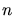is the number of cells per unit length,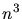switching operations are necessary for our assumptions. Fortunately this has to be done only once per time-step. Suppose a grid of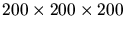cells. Switching all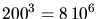cells does not really take a long time on state-of-the-art processors running at clock frequencies of 500MHz (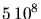instructions per second). This switching of the indices is not the limiting factor. Moreover it is common to all three methods and therefore omitted in the following considerations.

Before switching the marked cells it is necessary to decide which ones have to be marked. This is done with the structuring element applied at each surface cell. Basically, there are about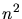surface cells needing scanning operations in their vicinity. Using the original implementation it has to be tested for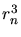cells in the vicinity of a surface cell whether they are less than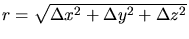away from the considered surface cell.is the local etch or deposition rate which determines the extension of the structuring element and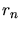is the diameter of the structuring element given as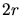in number of cells. This makes in sum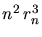operations. For the assumed 200 cells per unit length and a rateresolved by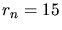cells this makes already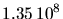operations. These are almost 20 times more operations than necessary for the index-switching.

For the spherical segment method the number of scanning operations is reduced fromto simply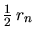for line elements at surface cells in a plane parallel to the planes of the coordinate system, to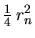for two-dimensional quarter-circle elements at edge cells, and to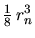for arbitrary located cells. Here enters the complexity of the geometry resulting in a combined overall exponent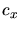depending on the ratio between plane, edge, and corner cells contained in the actual structure. The overall equation for the number of operations derives to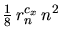, with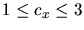. The prefactor of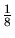is kept, sincehas the biggest influence on the overall acceleration. Again, for the worst case the acceleration factor is 8 (eights of spheres instead of spheres) with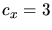. With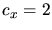, which is a very cautious assumption, the number of operations is reduced to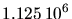, which is less than the number of index switching operations and approximately 120 times less than theoperations needed for scanning the complete spheres. With a rough estimation the benchmark example rather suggests an exponent of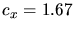for the first time-step on the initial structure which consists only of vertical and horizontal planes with sharp corners and of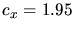for the second, already more complex geometry including curved regions. (2b) in Table 2.1 is about 125 times faster than (1b) for. This is in very good agreement with the factor of 120 derived above forassumed as 2. It explains that the algorithm gives an acceleration in the range of two orders of magnitude and that the second time-step takes twice as long as the first one, which is due to the more complex geometry for the second time-step, resulting in a higher exponent.

Now let's consider the situation for the line algorithm. Again there aresurface cells, but now onlyoperations are needed for the linear structuring elements applied at each surface cell. Still, two terms are missing for this case. Firstly, this method needs interpolation at convex corners. For each surface cell located at a convex corner about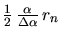interpolation operations are necessary in the implementation used for this comparison. This includes theoperations from above.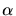is the angle between the surface normals of two planes forming a convex corner andis the angular step width of the interpolation necessary to maintain a smooth surface. The second factor is the calculation of the surface normals, which requires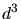operations for summing up the vectors of the cube face normals in the vicinity of the surface cells. This makes in sum about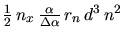operations ifis assumed to be the ratio between convex surface cells and the total number of surface cells. For the numerical assumptions of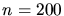,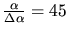,resolved bycells,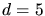, and the time needed for the simulations taken from Table 2.1,can be estimated as 0.15 for both time-steps by a simple division assuming a constant time per operation throughout all simulations.

Taking a look at Fig. 2.6 clearly reveals, thatcannot be similar in the two time-steps as derived in the above estimation. For the first time step only the upper rim of the cylindrical via has convex surface cells with two exposed cube faces, whereas for the second time-step, all surface cells forming the curvature around the initial rim have to be considered as convex corners in terms of surface cells. In the latter case the bottom and sidewall areas of the feature become smaller, which saves linear operations without interpolation and screens the proper calculation of the ratio. The reason therefore is the simplified assumption ofsurface cells, which does not consider vertical sidewalls and curved regions. Additionally, this simplified assumption explains, why this algorithm shows less dependence on the complexity of the structure, since the increasing number of convex corner cells is partially compensated by the shrinking size of the feature. However, the derivation of the required number of operations is applicable to the estimation of the acceleration which can be gained with this method.

A further remark is necessary for the line element algorithm. It turned out, that the linear structuring elements imply two contradicting requirements. For minimizing the discretization error, the lines are always applied at the center of an exposed cube surface, whereas for representing the correct growth direction they have to be applied in the center of the surface cell. Since only one origin of the structuring element is possible, this leads to the consequence, that the final geometry obtained with this method slightly differs from the results from the previous methods. The discrepancy prevents the method from being applied to isotropic or anisotropic models. Nevertheless, the line algorithm is used in our cellular topography simulator, namely, for cases where the rate vector is not given by the surface normal but by the directionality inherent to the process to be modeled. Line elements are therefore implemented for unidirectional etching and deposition as well as for sputter deposition where the rate is given as vector integral of the particle distribution function over the visible solid angle. A detailed explanation of this implementation will be given in Chapter 6.

With regard to CPU time, the surface orientation dependent linear element algorithm could be the method of choice if additional surface information is given, e.g., in a triangular format, and has not to be calculated internally with high computational costs. In this way thedependence could be discarded from the estimation of the number of required operations. Considering overall performance taking into account simulation time, accuracy, and the lack of information about surface orientation, the spherical segment algorithm is suited best for the application to isotropic etching and deposition steps within the cellular data representation. Nevertheless, linear structuring elements find application where the direction of the lines is not determined by the surface normal but by the directionality of the etching or deposition process, which is the case for unidirectional etching and deposition (cf. Section 3.2.3) and for sputter deposition (cf. Section 6.1.5).

These considerations conclude the introduction of the cellular structuring element approach and its recent developments. How the improved algorithms are applied to different models for etching and deposition processes will be demonstrated later in this thesis. Basic models will be found in Section 3.2, the description of more complex applications starts in Chapter 6. However, before starting etching and deposition simulations we need some input geometries on which to apply the processes, i.e., we need some silicon and a method to apply patterns onto the wafers. This is absolutely necessary in order to obtain selective etching and deposition forming three-dimensional structures on the wafer and needed for the simulation in the same way as for manufacturing of the desired devices. So let's first address the generation of three-dimensional geometries.

W. Pyka: Feature Scale Modeling for Etching and Deposition Processes in Semiconductor Manufacturing